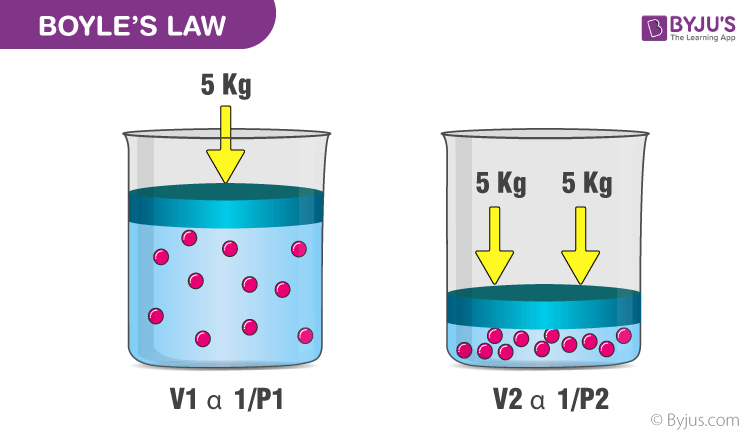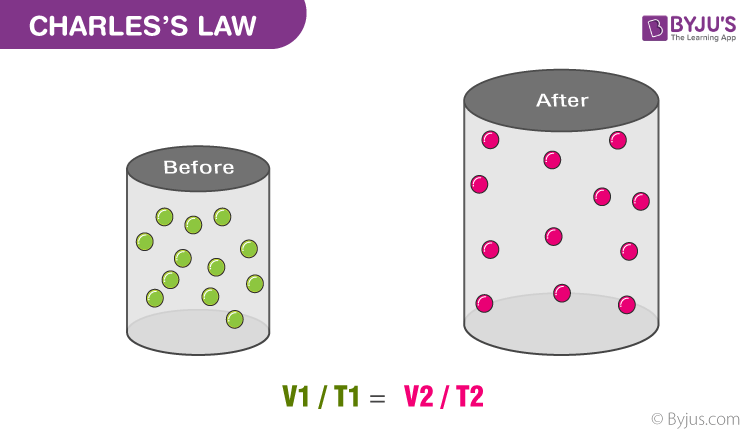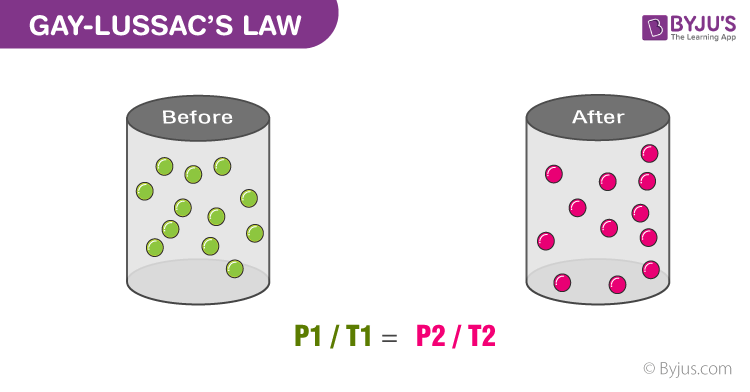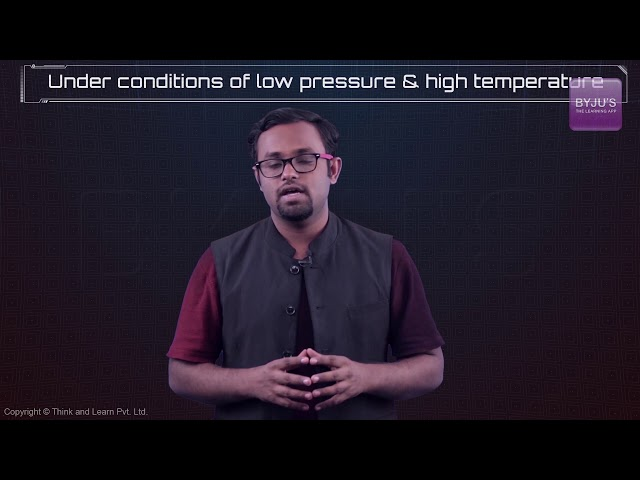Know more about the Repeaters Batch Know more about the Repeaters Batch

# The Gas Laws

## Introduction: What are the Gas Laws?

The gas laws are a group of laws that govern the behaviour of gases by providing relationships between the following:

• The volume occupied by a gas.
• The pressure exerted by a gas on the walls of its container.
• The absolute temperature of the gas.
• The amount of gaseous substance (or) the number of moles of gas.

The gas laws were developed towards the end of the 18th century by numerous scientists (after whom, the individual laws are named). The five gas laws are:

• Boyle’s Law, which provides a relationship between the pressure and the volume of a gas.
• Charles’s Law, which provides a relationship between the volume occupied by a gas and the absolute temperature.
• Gay-Lussac’s Law, which provides a relationship between the pressure exerted by a gas on the walls of its container and the absolute temperature associated with the gas.
• Avogadro’s Law, which provides a relationship between the volume occupied by a gas and the amount of gaseous substance.
• The Combined Gas Law (or the Ideal Gas Law), which can be obtained by combining the four laws listed above.

Under standard conditions, all gasses exhibit similar behaviour. The variations in their behaviours arise when the physical parameters associated with the gas (such as temperature, pressure, and volume) are altered. The gas laws basically describe the behaviour of gases and have been named after the scientists who discovered them.

We will look at all the gas laws below and also understand a few underlying topics.

## Boyle’s Law

Boyle’s law gives the relationship between the pressure of a gas and the volume of the gas at a constant temperature. Basically, the volume of a gas is inversely proportional to the pressure of a gas at a constant temperature.Boyle’s law equation is written as:

V ∝ 1/P

Or

P ∝ 1/V

Or

PV = k1

Where V is the volume of the gas, P is the pressure of the gas and K1 is the constant.  Boyle’s Law can be used to determine the current pressure or volume of gas and can be represented also as;

P1V1 = P2V2

### Boyle’s Law-Related Problem

An 18.10mL sample of gas is at 3.500 atm. What will be the volume if the pressure becomes 2.500 atm, with a fixed amount of gas and temperature?

Solution:

By solving with the help of Boyle’s law equation

P1V1 = P2V2

V2 = P1V1 / P2

V2 = (18.10 * 3.500atm)/2.500atm

V2 = 25.34 mL

## Charle’s Law

Charle’s law states that at constant pressure, the volume of a gas is directly proportional to the temperature (in Kelvin) in a closed system. Basically, this law describes the relationship between the temperature and volume of the gas.Mathematically, Charle’s law can be expressed as;

V ∝ T

Where, V = volume of gas, T = temperature of the gas in Kelvin. Another form of this equation can be written as;

V1 / T1 = V2 / T2

### Charle’s law problem

A sample of Carbon dioxide in a pump has a volume of 21.5 mL and it is at 50.0 oC. When the amount of gas and pressure remain constant, find the new volume of Carbon dioxide in the pump if the temperature is increased to 75.0 oC.

Solution:

V2 = V1T2/T1

V= 7,485.225/ 323.15

V= 23.16 mL

## Gay-Lussac Law

Gay-Lussac law gives the relationship between temperature and pressure at constant volume. The law states that at a constant volume, the pressure of the gas is directly proportional to the temperature for a given gas.If you heat up gas, the molecules will be given more energy, they move faster. If you cool down the molecules, they slow down and the pressure decreases. The change in temperature and pressure can be calculated using Gay-Lussac law and it is mathematically represented as;

P ∝ T

Or

P / T = k1

or

P1 / T1 = P2 / T2

Where P is the pressure of the gas and T is the temperature of the gas in Kelvin.

### Gay-Lussac Law related problem

Determine the pressure change when a constant volume of gas at 2.00 atm is heated from 30.0 °C to 40.0 °C.

Solution:

P1 = 2.00 atm
P2 =?
T1 = (30 + 273) = 303 K
T2 = (40 + 273) = 313 K

According to the Gay-Lussac law
P ∝ T
P/T = constant
P1/T1 = P2/T2
P2 =( P1 T2 ) / T1
= (2 x 313) / 303
=2.06 atm

Avogadro’s law states that if the gas is an ideal gas, the same number of molecules exists in the system. The law also states that if the volume of gases is equal it means that the number of the molecule will be the same as the ideal gas only when it has equal volume. This above statement can be mathematically expressed as;

V / n = constant

Or

V1 / n1 = V2 / n2

Where V is the volume of an ideal gas and n in the above equation represent the number of gas molecules.

### Problems related to Avogadro’s Law

At constant temperature and pressure, 6.00 L of a gas is known to contain 0.975 mol. If the amount of gas is increased to 1.90 mol, what new volume will result?

Solution:

V1 = 6.00 L
V2 = ?
n1 = 0.975
n2 = 1.90 mol

V ∝ n
V/n = constant
V1 / n1 = V2 / n2
V2 = V1n2/n1
V2 = (6 x 1.90)/ 0.975 = 11.69 L

## Combined Gas Law

The combined gas law is also known as a general gas equation is obtained by combining three gas laws which include Charle’s law, Boyle’s Law and Gay-Lussac law. The law shows the relationship between temperature, volume and pressure for a fixed quantity of gas.

The general equation of combined gas law is given as;

PV / T = k

If we want to compare the same gas in different cases, the law can be represented as;

P1V1 / T1 = P2V2 / T

Also Read: Kinetic Theory of Gas

## Ideal Gas Law

Much like the combined gas law, the ideal gas law is also an amalgamation of four different gas laws. Here, Avogadro’s law is added and the combined gas law is converted into the ideal gas law. This law relates four different variables which are pressure, volume, no of moles or molecules and temperature. Basically, the ideal gas law gives the relationship between these above four different variables.

## Recommended Videos

### Boyle’s Law – Video Lesson### Ideal Gas EquationMathematically Ideal gas law is expressed as;

PV=nRT

Where,

V = volume of gas.

T = temperature of the gas.

P = pressure of the gas.

R = universal gas constant.

n denotes the number of moles.

We can also use an equivalent equation given below.

PV = kNT

Where, k = Boltzman constant and N = number of gas molecules.

## Ideal Gas

Ideal gases are also known as a perfect gas. It establishes a relationship among the four different gas variables such as pressure (P), Volume(V), Temperature(T) and amount of gas (n).

### Ideal Gas Properties and Characteristics

• The motion of ideal gas in a straight line constant and random.
• The gas occupies a very small space because the particle in the gas is minimal.
• There is no force present between the particle of the gas. Particles only collide elastically with the walls of the container and with each other.
• The average kinetic energy of the gas-particle is directly proportional to the absolute temperature.
• The gases are made up of many the same particles (atoms or molecules) which are perfectly hard spheres and also very small.
• The actual volume of the gas molecule is considered negligible as compared to the space between them and because of this reason they are considered as the point masses.

## Gas Law Formula Table

The following table consists of all the formulas of Gas Law.

 Gas Law Formula Description Charle’s Law V1/T1=V2/T2 At constant P, as the volume increases the temperature also increases. Boyle’s Law P1V1=P2V2 At constant T, if pressure increases then volume decreases. Gay- Lussac Law P1/T1=P2/T2 At constant V as pressure increases the temperature also increases. Avogadro’s Law V / n = constant When the amount of gas increases, the volume of the gas also increases. Ideal Gas Law PV=nRT

## Gas Law Problems

A sealed jar whose volume is exactly 1L which contains 1 mole of air at temperature 20-degree Celcius, assuming that the air behaves as an ideal gas. So what is the pressure inside the jar in Pa?

Solution:

By solving with the help of ideal gas equation

PV=nRT

(1) By rearranging the equation we can get,

P=nRT/V

(2) Write down all the values which are known in S.I unit

n= 1

R= 8.314J/K/mol

T= 20degree celcius=(20+273.15)K=293.15K

V=1L=0.001m3

(3) Put all the values in the equation

P= nRT/V

P=(1*8.314*293.15)/0.001

P= 2,437,249

P=2.437*10^6 Pa

The pressure is almost 24atm.

## Application Of Gas-law

During summer when the temperature is high and pressure is also high, a tire is at risk of bursting because it is inflated with air. Or when you start climbing a mountain you feel some problems related to inhaling? Why does it happen?

When the physical condition is changing with changing in the environment the behaviour of gases particle also deviates from their normal behaviour. These changes in gas behaviour can be studied by studying various laws known as gas law.

The gas laws have been around for quite some time now, and they significantly assist scientists in finding amounts, pressure, volume, and temperature when coming to matters of gas.

Besides, the gas law along with modern forms are used in many practical applications that concern a gas. For example, respiratory gas measurement of tidal volume and vital capacity etc are done at ambient temperature while these exchanges actually take place in the body at 37-degree Celcius. The law is also used often in thermodynamics as well as in fluid dynamics. It can be used in the weather forecast systems.

## Frequently Asked Questions on Gas Laws

### What is an ideal gas?

Gases are puzzling. They are packed with a large number of very energetic gas molecules that can collide and interact. Because it’s difficult to precisely characterise a real gas, the concept of an ideal gas was developed as an approximation to help us the model and understand the behaviour of real gases.

### What are the rules followed by ideal gas?

Ideal gas molecules are neither attracted nor repellent to one another. An elastic collision is the only interaction between ideal gas molecules when they collide with each other or with the container’s walls.

The volume of ideal gas molecules is zero. The Ideal gas molecules are considered as point particles with no volume in and of themselves.

### What is the expression for ideal gas law?

PV = nRT
P is the pressure of the ideal gas.
V is the volume of the ideal gas.
T is the temperature of the ideal gas.
R is the gas constant.
n is the number of moles.

Test Your Knowledge On Gas Laws!